##1. Projective Base

Here I work in the standard model of projective plane, consisting of the classes X= [x] = [x1, x2, x3] of points of R3 modulo non-zero multiplicative constants.
A projective base consists of four points of the plane {A, B, C, D} in general position.

We say that four points {A,B,C,D} are in general position when these points are represented by corresponding vectors {[a], [b], [c], [d]} of R3, such that any three out of {a,b,c,d} build a basis of R3.
The fourth point D, corresponding to vector d = k1a + k2b + k3c (*), is called the coordinator or unit point of the basis. It is used to fix the scalar multiples of vectors a, b, c (I often say "calibrate") with respect to which we define coordinates (below). Lines {[a],[b],[c]} define the directions and (*) is used to define the three vectors (up to multiplicative constant) by (parallel) projecting d on the three lines.

 Every projective base introduces a coordinate system in which we write X = uA + vB + wC, {u,v,w} being defined modulo a non-zero multiplicative constant, and resulting from the corresponding representetion of [x] = X, through the basis vectors: x = u(k1a) + v(k2b) + w(k3c). These systems of coordinates are discussed in ProjectiveCoordinates.html .
 In particular, points {A,B,C,D} have correspondingly the coordinates {(1,0,0), (0,1,0), (0,0,1), (1,1,1)}.

##2. Projective lines

Two different points {A,B} of the projective plane define a projective line consisting of all points X that can be expressed as X = pA + qB, for some numbers {p,q}.
If a point X can be expressed as a combination in two ways X = pA + qB and X = p'A' + q'B', this means that it belongs to both lines determined by the pairs {A,B} and {A',B'} hence it coincides with the intersection of these two lines.
In the standard model of projective plane, described above, projective lines consist of sets of euclidean lines all of which are contained in some euclidean plane passing through the origin. Such a plane is described by an equation of the form
px + qy + rz = 0.
Given {A=[a], B=[b]} the corresponding coefficients (p,q,r) are the coordinates of the vector product axb.
Inversely, given the equation of the plane and selecting two vectors {a,b} satisfying this equation (lying in the plane), all other vectors of that plane are combinations c = ua + vb of these two vectors.
The structure of projective lines independent from the surrounding plane is studied in ProjectiveLine.html .

##3. Harmonicity expressed in coordinates

Consider the complete quadrilateral drawn below, where {A,B,C,D} constitute a projective base. Then points {P, Q} are harmonic conjugate with respect to {A, B} and their coordinates are P = A + B and Q = A - B.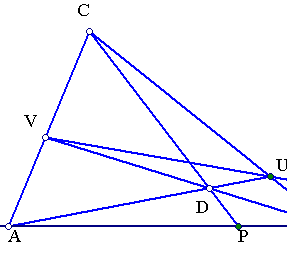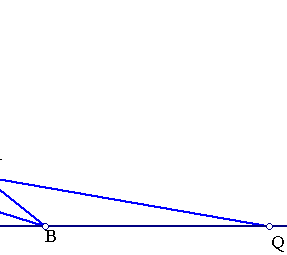Since P is on line CD, we have P = cC - dD = cC - d(A+B+C) = (c-d)C - dA - dB. Since P is on line AB it must be (c-d) = 0, hence P = A+B (coordinates defined modulo multiplicative constant!).
Thinking analogously for U and V, it must be U = C + B and V = C + A. Hence U-V = A - B = Q'. But Q' being both linear combination of {U,V} and {A,B}, must coincide with the intersection point of the corresponding lines i.e. Q' = Q.
More general the harmonic conjugate of a point P = sA+tB is Q = sA-tB. To see this consider again a point D on line CP, this time having coordinates D=C+dP=C+d(sA+tB), implying D-sdA = C+dtB. In this equation the left side represents a point on line DA and the right side represents a point on line CB hence both sides represent the intersection point U of these lines: U=D-sdA=C+dtB. Analogously D-tdB=C+dsA is the representation of V = D-tdB=C+dsA. From these we have U-V=(D-sdA)-(D-tdB)=tdB-sdA. Again U-V is a point on line UV and (tdB-sdA) is a point on line AB, hence coincides with Q. Thus, Q=d(tB-sA), which is equivalent with Q = sA-tB.

Remark In this paragraph I assumed that P, Q are harmonic conjugate with respect to A,B (see Harmonic.html ) and deduced their expression in terms of coordinates. One can also define the cross ratio in terms of coordinates, as I do it in the next paragraph.

##4. Cross-ratio in terms of line coordinates

Considering a line AB and a point C on it, C = xA + yB, we could try to define the ratio x/y as in euclidean geometry. This fails here however, since (x,y) depends on the vectors selected to represent points A=[a] and B=[b].
Passing though to the cross ratio of two points C = xA+yB and D = uA+vB with respect to {A,B} and defining it to be equal to (x/y):(u/v), we do find something meaningful. In fact, an easy calculation shows that if we change the representation of A[a], B[b], to A[a'], B[b'], and use the same definition: C = x'A+y'B, D = u'A+v'B, then the transition function from (u,v) to (u',v') is given by an invertible relation of the form x'=rx, y'=sy (if a'=ra, b'=sb), so that x'/y' = (x/y)(r/s). So the cross ratio (x/y):(u/v) is the same for the two representations of points A, B. Thus, it expresses a geometric relation of the four collinear points {A,B,C,D}, independent of their coordinate representation. This is made precise in ProjectiveLine.html .
Having this definition for the cross-ratio, points {C,D} are called harmonic conjugate with respect to {A,B}, when their cross-ratio (x/y):(u/v) = -1, implying x/y = -u/v. Thus we find again the result of (3), that the harmonic conjugate of C=xA+yB with respect to (A,B) is D=xA-yB.

##5. Desargues theorem in projective coordinates

Two point-perspective triangles ABC, A'B'C' are also line-perspective and vice versa.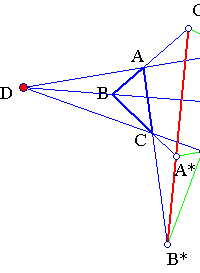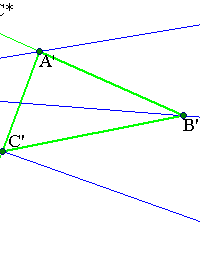Assume ABC, A'B'C' point-perspective i.e. lines AA', BB', CC' have a common point D. Then D can be written: D = uA + u'A' = vB + v'B' = wC + w'C'. Taken in pairs these equations are equivalent to:
(i) uA - vB = v'B' - u'A', (ii) wC-uA = u'A' - w'C', and (iii) vB - wC = w'C'-v'B'. Equation (i) expresses the intersection point C* of lines AB and A'B'. Analogously (ii) and (iii) express the intersection points B* and A* of lines AC, A'C' and BC, B'C'.
The three points satisfying C* + A* + B* = (uA-vB) + (vB-wC) + (wC-uA) = 0 are on a line.
 For the inverse, assume {A*, B*, C*} are collinear, and taking appropriate projective base, A* = B*+C*. We may assume that C* = uA - vB = v'B' - u'A' and B* = wC - u0A = u0'A' - w'C' ==> A*=B*+C*=uA-vB+wC-u0A, and since A* is on BC it must be (u-u0)=0. Analogously u'-u'0 = 0 and the intersection points of AA', BB', CC' coincide.
See Desargues.html for a standard geometric proof.

##6. Trilinear polar in projective coordinates

Consider a triangle ABC and a point D not on its sides. Let {A',B',C'} be the intersections of pairs of lines {(AD,BC), (BD,CA), (CD,AB)} respectively. Then the intersection points of line pairs {C''=(AB,A'B'), A''=(BC,B'C'), B''=(CA,C'A')} are on a line. This is the trilinear polar of point D with respect to the triangle ( TrilinearPolar.html ).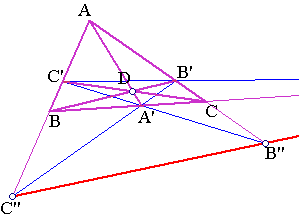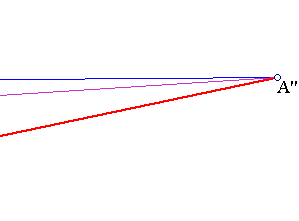By what we saw in the foregoing applications, taking the projective base {A,B,C,D}, points {A',B',C'} are represented correspondingly as {B+C, C+A, A+B}, and the harmonic conjugate to them {A''=B-C,B''=C-A,C''=A-B}. By adding the expressions we find that A''+B''+C''=0, proving the collinearity of the three points.

The applications indicate that incidence relations are very easily expressed and proved in the framework of projective geometry and with the aid of projective bases and their related coordinate system.